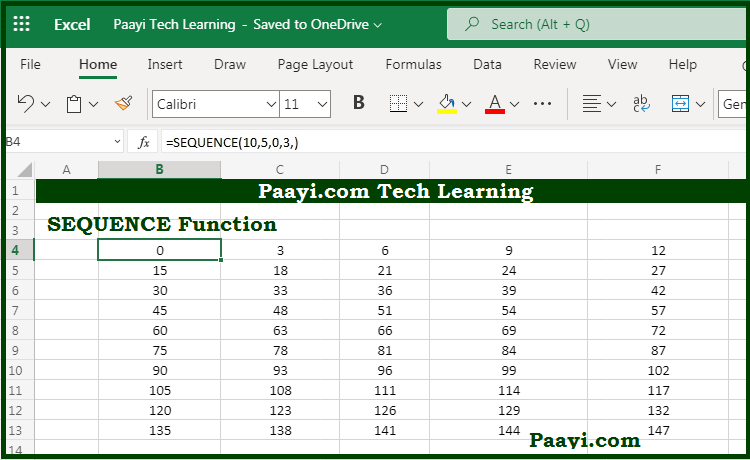# Learn How to Use Microsoft Excel SEQUENCE Function

Written by | 0 Comments | 741 Views

In this article, you will learn how to use the Microsoft Excel SEQUENCE function and its prime function in Microsoft Excel. You will also get to know the Microsoft Excel SEQUENCE function return value and syntax with the help of some examples.

Microsoft Excel SEQUENCE Function

The main function of the Microsoft Excel SEQUENCE function is to get an array of lists of sequential numbers. That means with the help of the SEQUENCE function; you can able to generate a list of sequential numbers in the array form. The array can be one or two-dimensional. Start and step values are used as an argument.

Return Value of SEQUENCE Function

The return value will be the array of sequential numbers.

Syntax of SEQUENCE Function

=SEQUENCE([rows], [columns], [start], [step])

Where the arguments:

rows: The number of rows to return.

columns: This is the number of columns to return (optional).

start: This is the starting value; default is 1 (optional).

step: This is the increment between each value, default is 1, (optional).

## How to Use Microsoft Excel SEQUENCE Function?As you know, with the help of the Microsoft Excel SEQUENCE function, you can able to generate a list of sequential numbers in the array form. The array can be one or two-dimensional, which you can control with the help of rows and columns arguments. Moreover, start and step value can also be provided as an argument.